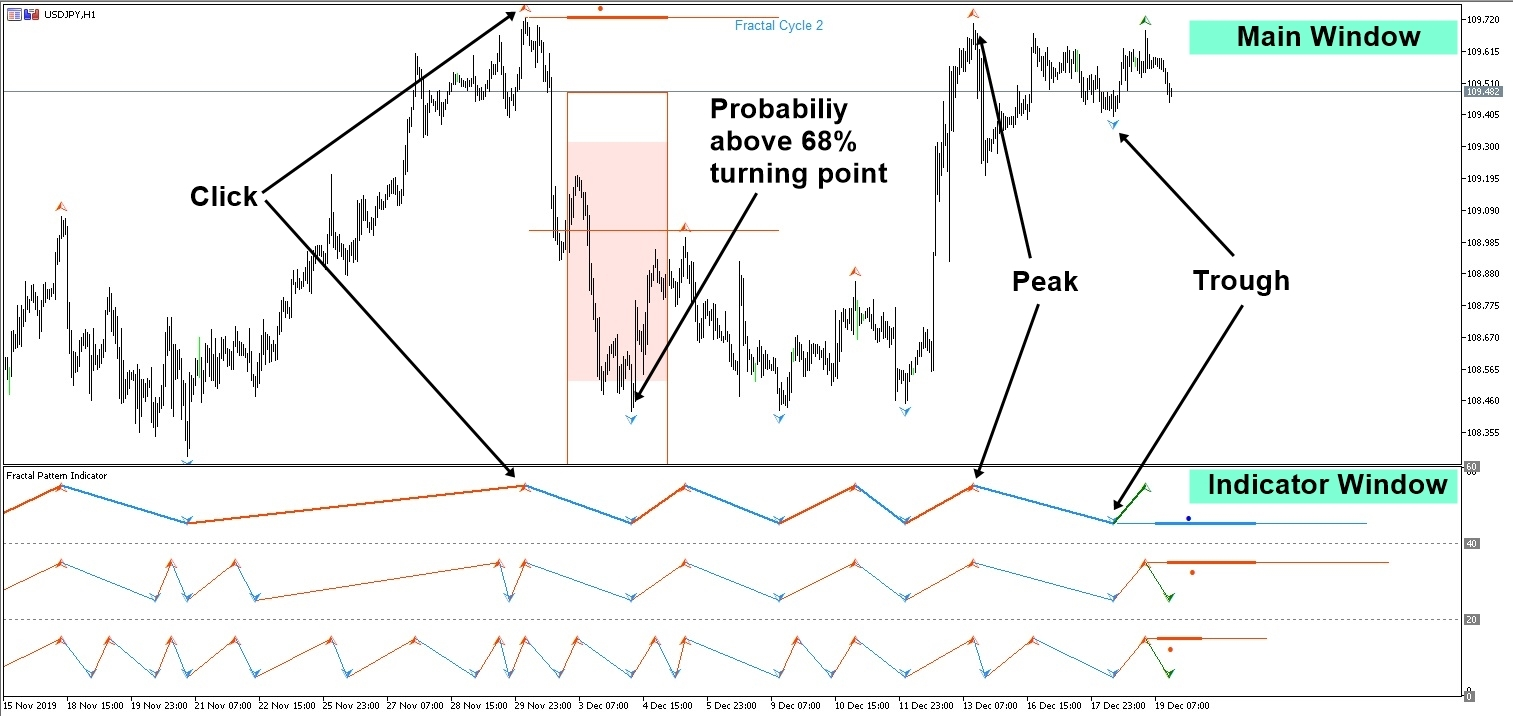# Probability in Price and Time from Fractal Pattern

Probability of price and time in Forex and Stock trading is the advanced information that can be used to determine a buy or sell position for your day trading. However, to understand how the probability of price and time in Forex and Stock market is structured, you need to understand the underlying dynamics in price and time as well as the crowd psychology around the financial market. Let us cover some of these aspects in in this article to improve your trading performance.

If the price data in forex and stock market possess the deterministic cycle, the algorithm like Fourier transformation, Wavelet transformation or Singular Spectrum Analysis could provide us the wavelength and amplitude of the specific cycle we want to trade. This could make our trading much easier and profitable. However, forex and stock market are affected by too many internal and external variables. The market are rather impulsive and fuzzy. In another words, the amplitude and wavelength of a cycle can not be guaranteed. Therefore, the deterministic cycle approach might be not the viable option for the choice of trading strategy.

Fractal wave in the price data possess the statistical self-similarity. This is close to the stochastic cycle rather than the deterministic cycle. In the stochastic cycle, the wavelength and amplitude of a cycle are not fixed but they can vary.The peak trough transformation introduced in this book is the effective method to extract the stochastic cycle in the financial market. In another words, we can measure the probability of a cycle after the price data is transformed to the peaks and troughs. Although we are likely to have the triangular fractal wave, this is hardly not a problem. We can still calculate the probability of the wavelength and amplitude of the specific cycle.

In real world, the price data will show complex structure, which the triangular fractal wave is combined with some trends. Hence, they are not stationary. We have shown the combined data structure of the fractal wave and a trend in the fifth column of the price pattern table. We can predict when a peak or trough will arrive in the price data with a probability. This was referred to as the turning point probability in the book: Predicting Forex and Stock Market with Fractal Pattern (Young Ho Seo, 2020). For example, when price is moving up from the trough, we can make the prediction when the peak will arrive by measuring the change in price and time. In another words, we can predict the position of swing high from the previous swing low in the statistical sense.

Likewise, when price is moving down from the peak, we can make the prediction when the trough will arrive by measuring the change in price and time. In another words, we can predict the position of swing low from the previous swing high in the statistical sense. This turning point probability is the statistical prediction made after the peak trough transformation of the price data. Since there are multiple of fractal wave cycles in the price data, we can calculate the turning point probability for each fractal wave cycle. In the book: Predicting Forex and Stock Market with Fractal Pattern (Young Ho Seo, 2020), the technique was referred to as the Fractal Cycle Analysis or FCA. Just like the Fourier transformation, Wavelet transformation or Singular Spectrum Analysis provide the analysis over multiple deterministic cycles, Fractal Cycle Analysis provides the analysis over multiple stochastic cycles. Fractal Cycle Analysis is the good technique to use when the deterministic cycle analysis is not applicable to the data.

Fractal Pattern Indicator is the first tool that helps you to measure the probability of turning point in price and time. In another words, you can predict the turning point using Fractal pattern indicator. Probability is good because it is measurable and quantifiable for your trading. We added some description of this probability in our product page below. However, it might be better to have some more explanation on this.

https://www.mql5.com/en/market/product/49170

https://www.mql5.com/en/market/product/49169